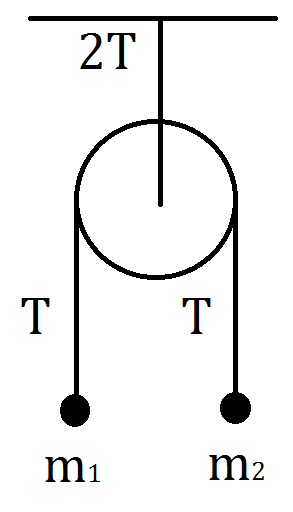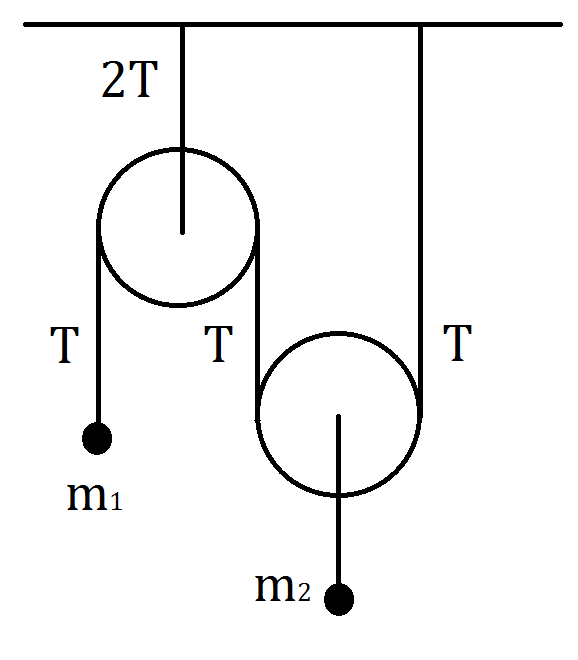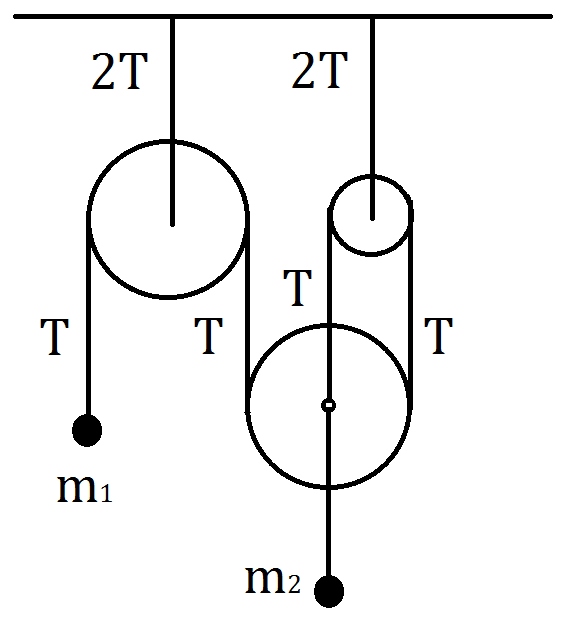# Atwood Machines

Back

Atwood Machines

1   Fixed Pulley

Let’s begin our analysis by studying the fundamental Atwood machine – a fixed pulley with two masses.Question

Find the accelerations of the masses and the tension in the string.

We solve problems involving Atwood machines by using F=ma equations and an equation of conservation of string.

F=ma equations$T-m_1g=m_1a_1$$T-m_2g=m_2a_2$

Conservation of String$a_1+a_2=0$

Accelerations$a_1=\frac{m_2-m_1}{m_1+m_2}g$$a_2=\frac{m_1-m_2}{m_1+m_2}g$

Tension$T=\frac{2m_1m_2g}{m_1+m_2}$

We can use the idea of effective mass of a fixed pulley to simplify our analysis in fixed pulley combinations.

Effective Mass of a Fixed Pulley$M=\frac{2T}{g}=\frac{4m_1m_2}{m_1+m_2}$

1.1   Fixed pulley combinationQuestion

Find the accelerations of the masses and the tension in the string.

F=ma equations$T-m_1g=m_1a_1$$T/2-m_2g=m_2a_2$$T/2-m_3g=m_3a_3$

We can use the idea of effective acceleration of a fixed pulley to simplify finding the equation for conservation of string in fixed pulley combinations.

Effective Acceleration of Fixed Pulley$A=\frac{a_2+a_3}{2}$

Conservation of String$a_1+\frac{a_2+a_3}{2}=0$

Accelerations$a_1=\frac{4m_2m_3-m_1(m_2+m_3)}{4m_2m_3+m_1(m_2+m_3)}g$$a_2=\frac{-4m_2m_3+m_1(3m_3-m_2)}{4m_2m_3+m_1(m_2+m_3)}g$$a_3=\frac{-4m_2m_3+m_1(3m_2-m_3)}{4m_2m_3+m_1(m_2+m_3)}g$

Tension$T=\frac{8m_1m_2m_3g}{2m_2m_3+m_1(m_2+m_3)}$

2   Free Pulley

Now let’s analyze systems with free pulleys in which they are not attached to supports.Question

Find the accelerations of the masses and the tension in the string.

F=ma equations$T-m_1g=m_1a_1$$2T-m_2g=m_2a_2$

Conservation of String$a_1+2a_2=0$

Accelerations$a_1=\frac{2m_2-4m_1}{4m_1+m_2}g$$a_2=\frac{2m_1-m_2}{4m_1+m_2}g$

Tension$T=\frac{3m_1m_2g}{4m_1+m_2}$

2.1$3$ StringsQuestion

Find the accelerations of the masses and the tension in the string.

F=ma equations$T-m_1g=m_1a_1$$3T-m_2g=m_2a_2$

Conservation of String$a_1+3a_2=0$

Accelerations$a_1=\frac{3m_2-9m_1}{9m_1+m_2}g$$a_2=\frac{3m_1-m_2}{9m_1+m_2}g$

Tension$T=\frac{4m_1m_2}{9m_1+m_2}g$

2.2$n$ Strings

We can generalize this to$n$ strings.

Question

Find the accelerations of the masses and the tension in the string.

F=ma equations$T-m_1g=m_1a_1$$nT-m_2g=m_2a_2$

Conservation of String$a_1+na_2=0$

Accelerations$a_1=\frac{nm_2-n^2m_1}{n^2m_1+m_2}g$$a_2=\frac{nm_1-m_2}{n^2m_1+m_2}g$

Tension$T=\frac{(n+1)m_1m_2}{n^2m_1+m_2}g$

2.3   Free Pulley In BetweenQuestion

Find the accelerations of the masses and the tension in the string.

F=ma equations$T-m_1g=m_1a_1$$2T-m_2g=m_2a_2$$T-m_3g=m_3a_3$

Conservation of String$a_1+2a_2+a_3=0$

Accelerations$a_1=\frac{3m_2m_3-m_1(m_2+4m_3)}{m_2m_3+m_1(m_2+4m_3)}g$$a_2=\frac{-m_2m_3+m_1(4m_3-m_2)}{m_2m_3+m_1(m_2+4m_3)}g$$a_3=\frac{-m_2m_3+m_1(3m_2-4m_3)}{m_2m_3+m_1(m_2+4m_3)}g$

Tension$T=\frac{4m_1m_2m_3}{m_2m_3+m_1(m_2+4m_3)}g$

2.4   Massless Free Pulley

Equivalence of Free PulleysThe subject of massless free pulleys is an interesting topic in Atwood machines. We can use a key idea derived here to solve more interesting problems.Question

Find the acceleration of the free pulley.

Procedure

Assume the free pulley has mass$m_2$. This is equivalent to the case with a free pulley derived above.$T-m_1g=m_1a_1$$2T-m_2g=m_2a_2$$a_1+2a_2=0$

We have$m_2=0$. The force on the pulley must be zero so$T=0$. We can use the first equation and third equation to solve for$a_2$. Note that we cannot use the second equation.$a_2=\frac{g}{2}$Question

Find the accelerations of the masses and the tension in the string.

Accelerations

Note that the net force on the left massless pulley must be zero.$a_1=-g$$a_2=-g$

Tension$T=0$

The techniques developed here can be applied to any Atwood Machine problem.